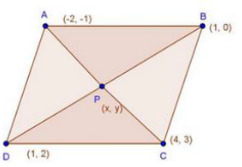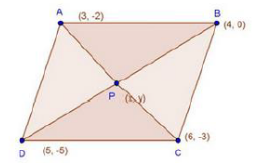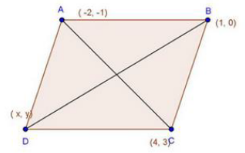# RD Sharma Class 10 Ex 14.3 Solutions Chapter 14 Coordinate Geometry

In this chapter, we provide RD Sharma Class 10 Ex 14.3 Solutions Chapter 14 Coordinate Geometry for English medium students, Which will very helpful for every student in their exams. Students can download the latest RD Sharma Class 10 Ex 14.3 Solutions Chapter 14 Coordinate Geometry pdf, Now you will get step by step solution to each question.

# Chapter 14: Coordinate Geometry Exercise – 14.3

### Question: 1

Find the coordinates of the point which divides the line segment joining (-1, 3) and (4, – 7) internally in the ratio 3 : 4.

### Solution:

Let P(x, y) be the required point.

Here, x1 = – 1

y1 = 3

x2  = 4

y2 = -7

m : n = 3 : 4

∴ The coordinates of P are (8/7, – 9/7)

### Question: 2

Find the points of trisection of the line segment joining the points:

(i) (5, – 6) and (-7, 5)

(ii) (3, – 2) and (-3, – 4)

(iii) (2, – 2) and (-7, 4)

### Solution:

(i) Let P and Q be the point of trisection of AB i.e., AP = PQ = QB

Therefore. P divides AB internally in the ratio of 1: 2, thereby applying section formula, the coordinates of P will be

Now, Q also divides AB internally in the ratio of 2:1 there its coordinates are

(ii) Let P, Q be the point of tri section of AB i.e. ,

AP = PQ = QB

Therefore, P divides AB internally in the ratio of 1: 2. Hence by applying section formula. Coordinates of P are

Now, Q also divides as internally in the ratio of 2: 1

So, the coordinates of Q are

Let P and Q be the points of trisection of AB i.e., AP = PQ = OQ

Therefore, P divides AB internally in the ratio 1:2. Therefore, the coordinates of P, by applying the section formula, are

Now. Q also divides AB internally in the ration 2 : 1. So the coordinates of Q are

### Question: 3

Find the coordinates of the point where the diagonals of the parallelogram formed by joining the points (-2, -1), (1, 0), (4, 3) and (1, 2) meet.

### Solution:Let P(x, y) be the given points.

We know that diagonals of a parallelogram bisect each other.

∴  coordinates of P are (1, 1)

### Question: 4

Prove that the points (3, 2), (4, 0), (6, -3) and (5, -5) are the vertices of a parallelogram.

### Solution:Let P(x, y) be the point of intersection of diagonals AC and 80 of ABCD.

Again,

Here mid-point of AC — Mid – point of BD i.e. diagonals AC and BD bisect each other.

We know that diagonals of a parallelogram bisect each other

∴ ABCD is a parallelogram.

### Question: 5

Three consecutive vertices of a parallelogram are (- 2, -1), (1, 0) and (4, 3). Find the fourth vertex.

### Solution:

Let A (-2,—1), B (1, 0), C (4, 3)and D (x, y) be the vertices of a parallelogram ABCD taken in order.Since the diagonals of a parallelogram bisect each other.

∴ Coordinates of the mid – point of AC = Coordinates of the mid-point of BD.

⇒ x + 1 = 2

⇒ x = 1

And,

Hence, fourth vertex of the parallelogram is (1, 2)

All Chapter RD Sharma Solutions For Class10 Maths

I think you got complete solutions for this chapter. If You have any queries regarding this chapter, please comment on the below section our subject teacher will answer you. We tried our best to give complete solutions so you got good